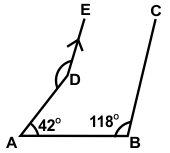# CREST Mathematics Olympiad Class 8 Sample Papers

REGISTER NOW

## Syllabus:

Section 1: Rational Numbers, Squares and Square Roots, Cubes and Cube Roots, Exponents and Powers, Comparing Quantities, Algebraic Expressions and Identities, Linear Equations in One Variable, Understanding Quadrilaterals, Constructions, Mensuration, Visualizing Solid Shapes, Data Handling, Direct and Inverse Variations, Factorization, Introduction to Graphs, Playing with Numbers.

Achievers Section: Higher Order Thinking Questions - Syllabus as per Section 1

Q.1

Match the statements of Column A with those of Column B:

 Column A Column B (1) The geometric point of a triangle which always lies inside the triangle (a) In centre (2) The geometric point of a triangle which always lies outside the triangle (b) Orthocentre (3) The geometric point of a triangle which always lies on two sides of the triangle (c) Circumcentre (4) The geometric point of a triangle which lies only on the longest side of the triangle (d) Excentre

 Q.2 Which of the following statements is correct?
 Q.3 In the given figure, DE||BC, ∠ABC = 118⁰, ∠DAB = 42⁰, then find the value of ∠ADE:Q.4 Out of the total 390 students studying in a college of  Arts and Science, boys and girls are in the ratio of 7 : 6 respectively and the number of students studying Arts and Science are in the ratio of 3 : 7 respectively. The boys and girls studying Arts are in the ratio of 4 : 5 respectively. How many boys are studying Science?
 Q.5 Consider the following statements: A number a1 a2 a3 a4 a5 a6 is divisible by 11 if 1. (a1 + a3 + a5) - (a2 + a4 + a6) = 02. (a1 + a3 + a5) - (a2 + a4 + a6) is divisible by 11 Which of these statements is/are correct?
 Q.6 What will be the factor of the following expression?625a12 - 81b12
 Q.7 If 2x-1 + 2x+1 = 320, then find the value of x:
 Q.8 Two poles, 18 m and 13 m high, stand upright in playground. If their feet are 12 m apart, then find the distance between their tops:
 Q.9 If Dennis is 1/3rd the age of his father Keith now, and was 1/4th the age of his father 5 years ago, then how old will his father Keith be 5 years from now?
 Q.10 The sides of a triangle are 5, 12 and 13 units. A rectangle of width 10 units is constructed whose area is equal to the area of the triangle. Find the perimeter of the rectangle:

Sample PDF of CREST Mathematics Olympiad for Class 8: